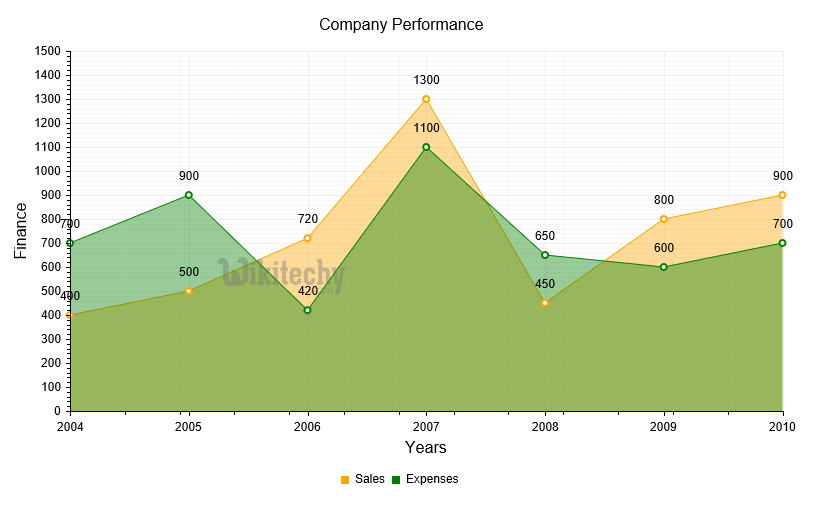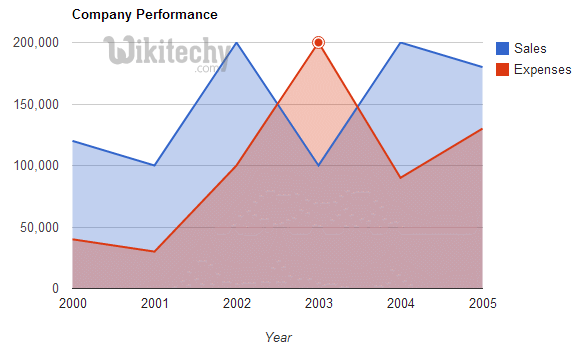# Google Charts - Google Charts tutorial - Google Charts Basic Area Chart - chart js - google graphs - google charts examples

## What is Basic Area Chart?

• The basic area chart is based on the line chart and the area between axis and line is filled with colors to indicate volume
• Basic Area charts emphasize the magnitude of change over time, and can be used to draw attention to the total value which is done across a trend.
• Basic area charts are used to see and compare the volume trend across time series
• Basic area charts are used for individual series representing a physically countable setLearn google charts - google charts tutorial - google charts basic area char - google charts examples - google charts programs

## Configuration

• Here the code which is given below shows us the configuration for basic area chart

## Sample code:

• The program which is given below shows us the full program of basic area chart which is used.Learn Google chart - Google chart tutorial - Google area chart - Google chart examples - Google chart programs